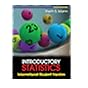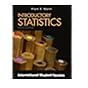Normal view MARC view ISBD view

# Introductory statistics / Prem S. Mann ; with contributions by Christopher Jay Lacke.

Call number: 519.5 M282I 2013 Material type:BookPublisher: Singapore : John Wiley & Sons, c2013. Edition: 8th ed., International student version.Description: xviii, 652,  p. : ill.ISBN: 9781118318706 (pbk.).
Contents:
Introduction -- Organizing and graphing data -- Numerical descriptive measures -- Probability -- Discrete random variables and their probability distributions -- Continuous random variables and the normal distribution -- Sampling distributions -- Estimation of the mean and proportion -- Hypothesis tests about the mean and proportion -- Estimation and hypothesis testing: two populations -- Chi-square tests -- Analysis of variance -- Simple linear regression -- Appendix A: Sample surveys, sampling techniques, and design of experiments -- Appendix B: Explanation of data sets -- Appendix C: Statistical tables.
Tags from this library: No tags from this library for this title. Log in to add tags.CL_General Book 519.5 M282I 2013 (Browse shelf) Available 1 1050035369CL_General Book 519.5 M282I 2013 (Browse shelf) Available 2 1050035370CL_General Book 519.5 M282I 2013 (Browse shelf) Available 3 1050035371CL_General Book 519.5 M282I 2013 (Browse shelf) Available 4 1050035372519.5 M282I 2011 Introductory statistics 519.5 M282I 2011 Introductory statistics 519.5 M282I 2011 Introductory statistics 519.5 M282I 2013 Introductory statistics 519.5 M282I 2013 Introductory statistics 519.5 M282I 2013 Introductory statistics 519.5 M282I 2013 Introductory statistics